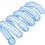# A Divergence Theorem Exercise

This problem was sent to me recently:

Verify the divergence theorem for the following force field and surface:

$\vec{F} = (4 x, -2 y^2, z^2) \\ S: x^2 + y^2 = 4 \\ 0 \leq z \leq 3$

Divergence theorem:

$\int \int \int \nabla \cdot \vec{F} \, dV = \int \int \vec{F} \cdot \vec{n} \, dS$

Vector field divergence and differential volume:

$\nabla \cdot \vec{F} = 4 - 4 y + 2 z \\ dV = dx \, dy \, dz$

Transforming to polar coordinates:

$\nabla \cdot \vec{F} = 4 - 4 r \, sin \theta + 2 z \\ dV = r \, dr \, d \theta \, dz$

Divergence integral (the triple integral is easy but tedious, so I've simply given the answer):

$\int_0^3 \int_0^{2 \pi} \int_0^2 (4 - 4 r \, sin \theta + 2 z) \, (r \, dr \, d \theta \, dz) \\ \int_0^3 \int_0^{2 \pi} \int_0^2 (4 r - 4 r^2 \, sin \theta + 2 r z) \, dr \, d \theta \, dz = 84 \pi$

As for the right side, it's obvious by inspection that the right-side integral over the $z = 0$ face is zero, and that the right-side integral over the $z = 3$ face is $36 \pi$. If the divergence theorem holds, the right-side integral over the side of the cylinder must equal $48 \pi$.

Cylinder side normal vector and vector field:

$\vec{n} = (cos \theta, sin \theta , 0) \\ \vec{F} = (4 r \cos \theta, -2 r^2 sin^2 \theta , z^2)$

Dot product and differential surface area:

$\vec{F} \cdot \vec{n} = 4 r \cos^2 \theta -2 r^2 sin^3 \theta \\ dS = r \, d \theta \, dz$

Over the cylinder side, $r = 2$, so:

$\vec{F} \cdot \vec{n} = 8 \cos^2 \theta - 8 sin^3 \theta \\ dS = 2 \, d \theta \, dz \\ \vec{F} \cdot \vec{n} \, dS = 16 (\cos^2 \theta - sin^3 \theta) \, d \theta \, dz$

Right-side integral over cylinder side:

$\int \int \vec{F} \cdot \vec{n} \, dS \\ \int_0^3 \int_0^{2 \pi} 16 (\cos^2 \theta - sin^3 \theta) \, d \theta \, dz \\ = 48 \int_0^{2 \pi} (\cos^2 \theta - sin^3 \theta) \, d \theta \\ = 48 \pi$Note by Steven Chase
3 years, 1 month ago

This discussion board is a place to discuss our Daily Challenges and the math and science related to those challenges. Explanations are more than just a solution — they should explain the steps and thinking strategies that you used to obtain the solution. Comments should further the discussion of math and science.

When posting on Brilliant:

• Use the emojis to react to an explanation, whether you're congratulating a job well done , or just really confused .
• Ask specific questions about the challenge or the steps in somebody's explanation. Well-posed questions can add a lot to the discussion, but posting "I don't understand!" doesn't help anyone.
• Try to contribute something new to the discussion, whether it is an extension, generalization or other idea related to the challenge.

MarkdownAppears as
*italics* or _italics_ italics
**bold** or __bold__ bold
- bulleted- list
• bulleted
• list
1. numbered2. list
1. numbered
2. list
Note: you must add a full line of space before and after lists for them to show up correctly
paragraph 1paragraph 2

paragraph 1

paragraph 2

[example link](https://brilliant.org)example link
> This is a quote
This is a quote
    # I indented these lines
# 4 spaces, and now they show
# up as a code block.

print "hello world"
# I indented these lines
# 4 spaces, and now they show
# up as a code block.

print "hello world"
MathAppears as
Remember to wrap math in $$ ... $$ or $ ... $ to ensure proper formatting.
2 \times 3 $2 \times 3$
2^{34} $2^{34}$
a_{i-1} $a_{i-1}$
\frac{2}{3} $\frac{2}{3}$
\sqrt{2} $\sqrt{2}$
\sum_{i=1}^3 $\sum_{i=1}^3$
\sin \theta $\sin \theta$
\boxed{123} $\boxed{123}$

Sort by:

We can also solve this by taking a projection on the XZ plane for the surface integral of the cylinder?

- 3 years, 1 month ago

In general, you can integrate over a projection instead of the actual surface. However, you have to formulate the integrand in a particular way when doing so. A vector calculus text book should have a description of that.

- 3 years, 1 month ago

Oh I see. Thank you!

- 3 years, 1 month ago